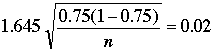SEARCH HOMEMath Central Quandaries & QueriesQuestion from Rae, a student: What sample size was needed to obtain an error range of 2% if the following statement was made? "75% of the workers support the proposed benefit package. These results are considered accurate to within + or - 2%, 18 out of 20 times." This seems like a straight forward question but I'm getting it wrong. Could you please help me out even just the set up would be appreciated so I can see if that's where I'm going wrong. ThanksHi Rae,

My understanding of this question is that someone has reported

"75% of the workers support the proposed benefit package. These results are considered accurate to within + or - 2%, 18 out of 20 times."

And you want to determine the sample size the researcher used. You know that 18 out of 20 is a 90% confidence interval, z0.05 = 1.645 and the sample proportion was 0.75 so the calculation to obtain an error bound of ± 0.02 wasSquaring both sides and solving for n gave me n = 1268.4.

HarleyMath Central is supported by the University of Regina and The Pacific Institute for the Mathematical Sciences.